# Natural equation

(diff) ← Older revision | Latest revision (diff) | Newer revision → (diff)

of a curve

A system of equations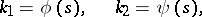defining the curvature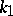and torsionof the curve as functions of the arc length parameteron the curve. For any regular functions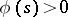and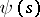there exists a curve, unique up to translation in space, with curvature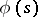and torsion. A necessary and sufficient condition for a curve to be in a plane is that its torsion vanishes identically. A necessary and sufficient condition for a curve to be a straight line (or a segment of a straight line) is that its curvature vanishes identically.

In the article above,must be positive in order to generate uniqueness of the curve; for existencesuffices (cf. [a1], Sects. 8.5.8 and 8.6.15).
Instead of "natural equation" one also finds the phrase "intrinsic equation of a curveintrinsic equation of a curve" . The representation of (certain special) plane curves by means of a relation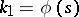goes back to L. Euler.